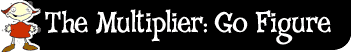﻿ The multiplier: go figure: doubling# Doubling

Another strategy is doubling. The idea behind this is that you double one of the numbers as many times as you need to work out the answer. It's really just using your 2 times table.

For example, let's look at 39 times 4. I double 39 to get 78.

This is the same as 2 times 39.

I double it again to get 156, which is 39 times 4.

Use this strategy to work out 16 times 4.

Doubling 16 gives?

Doubling 32 gives?

Did you get 64?

This strategy works best when you are multiplying by an even number.